### Variance/Standard Deviation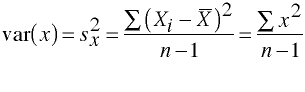### Standard Deviation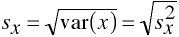### Covariance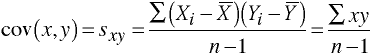### Another Look at Covariance

Consider the variance as being the covariance of a variable with itself.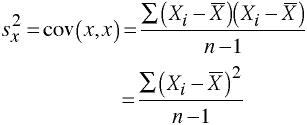Plotting Two Variables SimultaneouslyThe more tightly the points are clustered together the higher the correlation between the two variables and the higher the ability to predict one variable from another.

### Selected Scatter Plots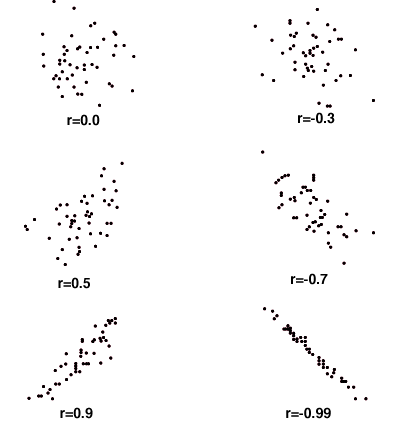### Pearson Product Moment Correlation Coefficient

Also known as, the Pearson correlation coefficient, or just the correlation coefficient.

Correlation coefficients can take on any value between -1 and +1, with + and - 1 representing perfect correlations between the variables. And a correlation of zero representing no relationship between the variables.

A rule of thumb for interpreting correlation coefficients:

``` Corr     Interpretation
0 to .1  trivial
.1 to .3  small
.3 to .5  moderate
.5 to .7  large
.7 to .9  very large```

Correlations are interpreted by squaring the value of the correlation coefficient. The squared value represents the proportion of variance of one variace that is shared with the other variable, in other words, the proportion of the variance of one variable that can be predicted from the other variable.

### Percent of Variance Accounted For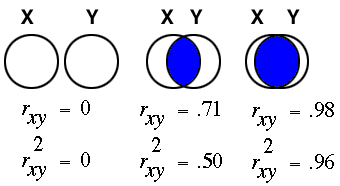### Correlation and Sample Size

The computation of correlation coefficients do not lend themselves to small sample sizes. The following table gives the recommended sample size for detecting various correlations with a power = 0.8 with an alpha = 0.05.
```corr   n
.10   617
.20   153
.30    68
.40    37
.50    22
.60    15
.70    10
.80     7
.90     5 ```

### Population Correlation Coefficient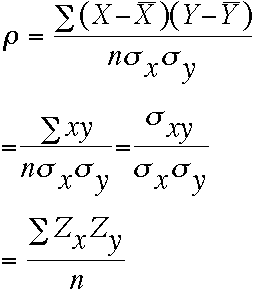### Sample Correlation Coefficient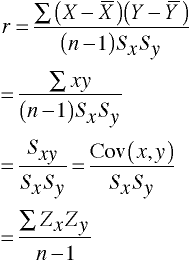Sources of Misleading Correlation Coefficients

• Restriction of Range
• Extreme Groups
• Combining Groups
• Outliers
• Curvilinearity

Restriction of Range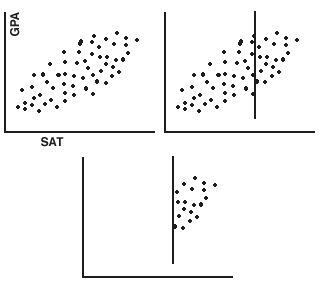Extreme Groups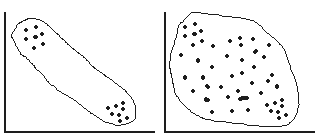Combining Groups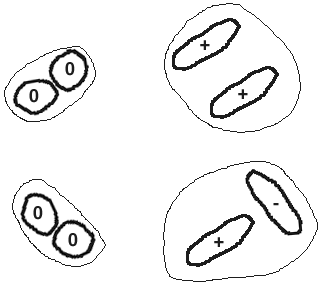Outliers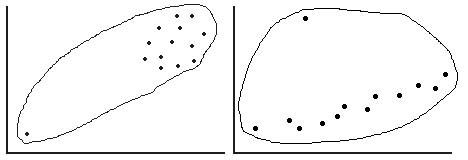Curvilinearity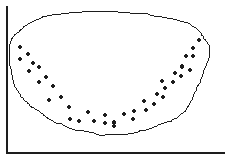Discuss Correlation & Causation

Of course, just because two variables are correlated it does not mean that they are causally related. Often a third variable, a lurking variable, that is not included in the analysis is responsible (causes) for the first two variables. A lurking variable is a variable that loiters in the background and affects both of the original variables

Other Correlation Coefficients

• Spearman rank-order correlation coefficient -- Spearman ρ
Used when data are ordinal. Interpreted like a Pearson correlation.

• Eta coefficient -- η
Indicates the degree of relationship between two variables even if the relationships is nonlinear.

• Eta-squared coefficient -- η2
Indicates how well one variable can be predicted from another even with nonlinear relationships. Interpreted in a manner similar to r2.

• Biserial correlation coefficient -- rbi
For use when one variable is continuous and the other is a dichotomous variable that reflects an underlying normal distribution.

• Point biserial coefficient -- rpb
For use when one variable is continuous and the other is a 'true' dichotomous variable.

• Phi coefficient -- φ
For use with two 'true' dichotomous variables. φ = (a*d - b*c)/sqrt(a*b*c*d)

• Tetrachoric correlation coefficient -- rtet
For use with two artificial dichotomous variables with underlying normal distributions.

• Multiple correlation coefficient -- Ra.bcd
The correlation between a and the set of variables b, c, and d.

• Squared multiple correlation coefficient - Coefficient of Determination -- R2a.bcd
The squared correlation between a and the set of variables b, c, and d. It represents the proportion of variability of a that is accounted for by the combination of b, c, and d.

• Partial correlation coefficient -- rab.c
The correlation between a and b with variable c partialed out. Partial correlations are useful in interpreting regression models.
Spearman's Rank Order Correlation

• A bivariate correlation for use when data are ranked data for both variables.
• Ranked data are scaled as ordinal data.
• Use Spearman's correlation, rs (ρ).

Spearman Example

 Sub xrank yrank d d2 a 1 3 -2 4 b 4 4 0 0 c 5 8 -3 9 d 10 5 5 25 e 8 2 6 36 f 14 15 -1 1 g 7 9 -2 4 h 2 6 -4 16 i 12 14 -2 4 j 9 7 2 4 k 15 13 2 4 l 3 1 2 4 m 13 12 1 1 n 11 10 1 1 o 6 11 -5 25 Sum 0 138Stata Example

```input xrank yrank
1  3
4  4
5  8
10  5
8  2
14 15
7  9
2  6
12 14
9  7
15 13
3  1
13 12
11 10
6 11
end

corr
(obs=15)

|    xrank    yrank
---------+------------------
xrank |   1.0000
yrank |   0.7536   1.0000
```

Another Stata Example

• Now, let's use Stata to create rank data and compare the Pearson correlation with the Spearman correlation.
```input y x
100 135
120 105
160 155
220 175
110 105
140 145
200 185
260 195
130 145
110 105
180 175
210 165
200 175
170 145
120 145
end

egen xrank = rank(x)

egen yrank = rank(y)

list

y          x      xrank      yrank
1.       100        135          4          1
2.       110        105          2        2.5
3.       110        105          2        2.5
4.       120        145        6.5        4.5
5.       120        105          2        4.5
6.       130        145        6.5          6
7.       140        145        6.5          7
8.       160        155          9          8
9.       170        145        6.5          9
10.       180        175         12         10
11.       200        185         14       11.5
12.       200        175         12       11.5
13.       210        165         10         13
14.       220        175         12         14
15.       260        195         15         15

corr x y xrank yrank
(obs=15)

|        y        x    xrank    yrank
---------+------------------------------------
y |   1.0000
x |   0.8768   1.0000
xrank |   0.9118   0.9853   1.0000
yrank |   0.9821   0.8753   0.9073   1.0000

spearman x y

Number of obs =      15
Spearman's rho =       0.9073

Test of Ho: x and y independent
Pr > |t| =       0.0000
```

Point Biserial Correlation

• A bivariate correlation for use when one variable is continuous and the other variable is a "true" dichotomous variable.

Point Biserial Example

```input y x
100 0
120 1
160 0
220 1
110 0
140 0
200 1
260 1
130 0
110 1
180 0
210 1
200 1
170 1
120 0
end

corr x y
(obs=15)

|        x        y
---------+------------------
x |   1.0000
y |   0.5541   1.0000
```

Fourfold Correlation - Phi Coefficient

• A bivariate correlation for use when both variables are dichotomous.

 Y 1 0 X 1 (a) 12 (b) 16 0 (c) 14 (d) 9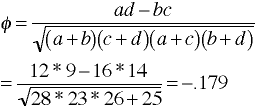Stata Example

• Use the dichotomous data with any Pearson correlation program and obtain the same correlation.
```input x y w
0 0 9
0 1 14
1 0 16
1 1 12
end

corr x y [fw=w]
(obs=51)

|        x        y
---------+------------------
x |   1.0000
y |  -0.1793   1.0000
```

• Or, use the tabulate command.
```tab x y [fw=w], all

|           y
x |         0          1 |     Total
-----------+----------------------+----------
0 |         9         14 |        23
1 |        16         12 |        28
-----------+----------------------+----------
Total |        25         26 |        51

Pearson chi2(1) =   1.6394   Pr = 0.200
likelihood-ratio chi2(1) =   1.6495   Pr = 0.199
Cramer's V =  -0.1793
gamma =  -0.3494  ASE = 0.252
Kendall's tau-b =  -0.1793  ASE = 0.138
```

When analyzing two-by-two tables, the value of Cramer's V is actually phi. Cramer's V is a generalization of the phi coefficient that can be used in tables larger than two-by-two.

Phil Ender, 15Jan98